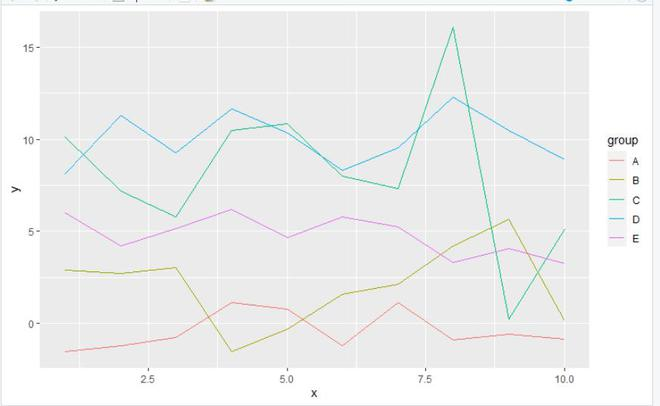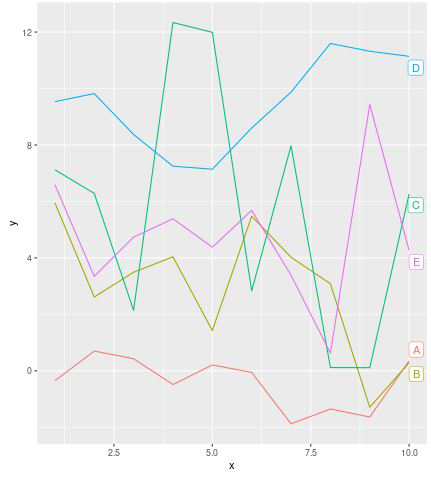Open in App
Not now

# Plot labels at end of ggplot line graph in R

• Last Updated : 14 Sep, 2021

In this article, we will be looking at the approach to plot labels at the end of the ggplot2 line plot in the R programming language.

In this approach to plot the labels at the end of the ggplot2 line, the user needs to install and import the ggplot2 and ggrepel package on the R working console, here the ggplot2 package will be used to plot the simple ggplot2 line pot and the ggrepel package is used here to add the labels at the end of the line plot plotted and then the user needs to call the geom_label_repel() function from ggrepel package with the theme function from ggplot2 package and specify its parameters are per the requirements, further this process will lead to the plotting to the labels at the end of the ggplot2 line plot in the R programming language.

geom_label_repel() function is used to adds text directly to the plot. geom_label_repel draws a rectangle underneath the text, making it easier to read. The text labels repel away from each other and away from the data points.

Syntax:

geom_label_repel( mapping = NULL,data = NULL,stat = “identity”,position = “identity”,parse = FALSE, nudge_x = 0,na.rm)

Parameters:

• mapping:-Set of aesthetic mappings created by aes or aes_. If specified and inherit.aes = TRUE (the default), is combined with the default mapping at the top level of the plot. You only need to supply mapping if there isn’t a mapping defined for the plot.
• data:-A data frame. If specified, overrides the default data frame defined at the top level of the plot.
• stat:-The statistical transformation to use on the data for this layer, as a string.
• position: -Position adjustment, either as a string, or the result of a call to a position adjustment function.
• parse:-If TRUE, the labels will be parsed into expressions and displayed as described in plot math
• na.rm:-If FALSE (the default), removes missing values with a warning. If True silently removes missing values.

Let us first plot a regular plot without any change, so that the difference is apparent.

Example: initial plot

## R

 `library``(``"ggplot2"``)``library``(``"ggrepel"``)`` ` `gfg<- ``data.frame``(x = 1:10,``                 ``y = ``c``(``rnorm``(10),``                       ``rnorm``(10,3,3),``                       ``rnorm``(10, 5, 3),``                       ``rnorm``(10, 10, 1.5),``                       ``rnorm``(10, 5, 2)),``                 ``group = ``rep``(``LETTERS``[1:5], each = 10))`` ` `ggplot``(gfg, ``aes``(x, y, col = group)) +``geom_line``()`

Output:Now let us apply the required according to the above approach to add plot labels at the end.

Example: Adding plot labels at the end

## R

 `library``(``"ggplot2"``)``library``(``"ggrepel"``)`` ` `gfg<- ``data.frame``(x = 1:10,``                 ``y = ``c``(``rnorm``(10),``                       ``rnorm``(10,3,3),``                       ``rnorm``(10, 5, 3),``                       ``rnorm``(10, 10, 1.5),``                       ``rnorm``(10, 5, 2)),``                 ``group = ``rep``(``LETTERS``[1:5], each = 10))`` ` `gfg\$label <- ``NA`` ` `gfg\$label[``which``(gfg\$x == ``max``(gfg\$x))] <- gfg\$group[``which``(gfg\$x == ``max``(gfg\$x))]`` ` `ggplot``(gfg, ``aes``(x, y, col = group)) +``geom_line``() + ``geom_label_repel``(``aes``(``  ``label = label), nudge_x = 1, na.rm = ``TRUE``) `

Output:My Personal Notes arrow_drop_up